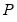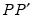fiml
Estimation by full information maximum likelihood.
fiml estimates a system of equations by full information maximum likelihood (assuming a multivariate normal distribution).
Syntax
system_name.fiml(options)
Options

 rcov = arg Restricted residual covariance matrix in estimation: “diag” (non-zero diagonal and zero off-diagonal elements), “usercov” (fully specified user covariance matrix), “userfactor” user provides the matrix, such thatequals the fully specified covariance matrix).Note that system objects estimated using a restricted FIML estimator are not backward compatible with earlier versions of EViews, and will be dropped from the workfile if opened in a version prior to 9.5. rcovname= arg Name of the matrix for determining the user specified residual covariance matrix.(Applicable when “rcov=usercov” or “rcov=userfactor”.) optmethod = arg Optimization method: “bfgs” (BFGS); “newton” (Newton-Raphson), “opg” or “bhhh” (OPG or BHHH), “legacy” (EViews legacy).“bfgs” is the default for new equations. optstep = arg Step method: “marquardt” (Marquardt - default); “dogleg” (Dogleg); “linesearch” (Line search).(Applicable when “optmethod=bfgs”, “optmethod=newton” or “optmethod=opg”.) b Use Berndt-Hall-Hall-Hausman (BHHH) as maximization algorithm. The default is Marquardt.(Applicable when “optmethod=legacy”.) cov=arg Covariance method: “ordinary” (default method based on inverse of the estimated information matrix), “huber” or “white” (Huber-White sandwich method). covinfo = arg Information matrix method: “opg” (OPG); “hessian” (observed Hessian), “(Applicable when non-legacy “optmethod=” with “cov=ordinary”.) m=integer Maximum number of iterations. c=number Set convergence criterion. The criterion is based upon the maximum of the percentage changes in the scaled coefficients. The criterion will be set to the nearest value between 1e-24 and 0.2. numericderiv / ‑numericderiv [Do / do not] use numeric derivatives only. If omitted, EViews will follow the global default. fastderiv / ‑fastderiv [Do / do not] use fast derivative computation. If omitted, EViews will follow the global default. showopts / ‑showopts [Do / do not] display the starting coefficient values and estimation options in the estimation output. prompt Force the dialog to appear from within a program. p Print estimation results.
Examples
sys1.fiml
estimates SYS1 by FIML using the default settings.
sys1.fiml(rcov=diag)
estimates SYS1 by FIML with the off-diagonal residual covariances set to zero.
sys1.fiml(rcov=user, rcovname=mycov)
estimates a FIML model using MYCOV as the residual covariance matrix.
Cross-references
See “System Estimation” for a discussion of systems in EViews.